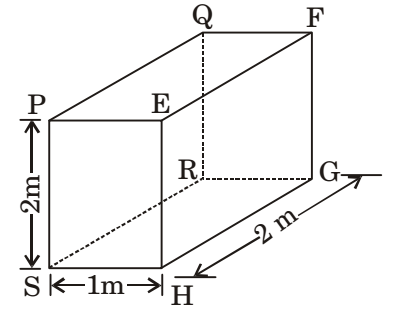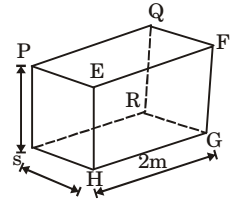## Heat Transfer Miscellaneous

#### Heat-Transfer

Direction: An un-insulated air conditioning duct of rectangular cross section 1 m × 0.5 m, carrying air at 20°C with a velocity of 10 m/s, is exposed to an ambient of 30°C. Neglect the effect of duct construction material. For air in the range of 20 – 30°C, data is as follows: thermal conductivity = 0.025 W/mK; viscosity = 18 μPa.s; Prandtl number = 0.73; density =1.2 kg/m3. For laminar flow Nusselt number is 3.4 for constant wall temperature conditions and, for turbulent flow, Nu = 0.023 Re0.8Pr0.33

1. The heat transfer per meter length of the duct, in watts, is

1. As Re > 4000, the flow is turbulent
Nu = 0.023 Re0.8Pr0.33
hL/k = 0.023 × (4.44 × 105)0.8 × (0.73)0.33
h × 0.0667/0.25 = 683.173
h = 25.60 W/m2K
Surface area of duct
A = 2 × al + 2 × bl
where, l = length of duct
∴ A = 3l
Heat transfer rate, Q = h A(T0 – T)
Q = 25.60 × 3l × (30 – 20)
Q/l = 769W

##### Correct Option: D

As Re > 4000, the flow is turbulent
Nu = 0.023 Re0.8Pr0.33
hL/k = 0.023 × (4.44 × 105)0.8 × (0.73)0.33
h × 0.0667/0.25 = 683.173
h = 25.60 W/m2K
Surface area of duct
A = 2 × al + 2 × bl
where, l = length of duct
∴ A = 3l
Heat transfer rate, Q = h A(T0 – T)
Q = 25.60 × 3l × (30 – 20)
Q/l = 769W

1. The Reynolds number for the flow is

1.  Lc = 4A = 4 × 1 × 0.5 = 0.666 D 2 × 1.5

Reynolds number,
 Re = ρLcV = 1.2 × 0.66 × 10 = 4.4 × 105 . μ 18 × 10-6

##### Correct Option: C

 Lc = 4A = 4 × 1 × 0.5 = 0.666 D 2 × 1.5

Reynolds number,
 Re = ρLcV = 1.2 × 0.66 × 10 = 4.4 × 105 . μ 18 × 10-6

1. Consider a laminar boundary layer over a heated flat plate. The free stream velocity is U. At some distance x from the leading edge the velocity boundary layer thickness is δv and the thermal boundary layer thickness is δT. If the Prandtl number is greater than 1, then

1.  Prandtl number = Molecular diffusivity of momentum Molecular diffusivity of heat

From question, since prandtl number > 1
∴ Velocity boundry thickness (δv) > thermal boundary thickness (δt)

##### Correct Option: A

 Prandtl number = Molecular diffusivity of momentum Molecular diffusivity of heat

From question, since prandtl number > 1
∴ Velocity boundry thickness (δv) > thermal boundary thickness (δt)

1. For the three-dimensional object shown in the figure below, five faces are insulated. The sixth face (PQRS), which is not insulated, interacts thermally with the ambient, with a convective heat transfer coefficient of 10W/m2K. The ambient temperature is 30°C. Heat is uniformly generated inside the object at the rate of 100 W/m3. Assuming the face PQRS to be at uniform temperature, its steady state temperature is1. Given data;
ho = W/m2K
ho = 30 °C
qG = 100 W/m3
Volume, V = 2×1×2=4 m3Heat generated, Q = qG × V = 100 × 4 = 400 W
Convection heat transfer from face PQRS,
Q = ho A (Ts – To)
400 = 10 × 2 × 2 (Ts – 30)
Ts = 40°C

##### Correct Option: D

Given data;
ho = W/m2K
ho = 30 °C
qG = 100 W/m3
Volume, V = 2×1×2=4 m3Heat generated, Q = qG × V = 100 × 4 = 400 W
Convection heat transfer from face PQRS,
Q = ho A (Ts – To)
400 = 10 × 2 × 2 (Ts – 30)
Ts = 40°C

1. Heat transfer coefficients for free convection in gases, forced convection in gases and vapours, and for boiling water lie, respectively, in the range of

1. 5-15; 20-200 and 3000-50000 W/m2K

##### Correct Option: A

5-15; 20-200 and 3000-50000 W/m2K